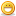# Thread: A simple program with a tough logic!!!

1. ## A simple program with a tough logic!!!

hi everyone

this question came to my mind and has troubling me now for almost a month....
so guys plz help me on this!!!

the program is very simple. in it a user has to enter a no. and what he gets the output is the same no. but in words instead of figures i.e. the output is somewhat like as follows:

Enter a no.:1121
The no. is:One thousand one hundred and twenty one

now that's what i want!!! and if anybody does this for me i'll be highly obliged!!!!

so guys i m waiting for ur answers!!!Reply With Quote

2. Take this as a start:

Number to Text Demo [VB5]
This demonstration program shows how to convert numbers to English as you might see in a check writing program. For example, 12345.67 is converted to "Twelve thousand, three hundred forty-five and 67/100".

 http://www.softcircuits.com/sw_vbsrc.htmReply With Quote

3. i just forgot to mention the main point!
the point is i wanna do this using C/C++
i m sorry again!Reply With Quote

4. A simple solution whould be to break down your original number in digits, and parse those digits one by one, using a switch/case-statement. In psuedo, you'd get something like this:

Code:
```var \$number = 1234
var \$thousands = 1
var \$hundreds = 2
var \$tens = 3
var \$ones = 4

switch (\$thousands)
{
case: 1:  \$thousandstring = "One thousand "; break;
case: 2:  \$thousandstring = "Two thousand "; break;
case: 3:  \$thousandstring = "Three thousand "; break;
// etc
}

switch (\$hundreds)
{
case: 1:  \$hundredsstring = "One hundred "; break;
case: 2:  \$hundredsstring = "Two hundred "; break;
// etc
}

// do the same for the tens and ones

// finally, concat the four strings you got:
\$result = \$thousandstring + \$hundredsstring + \$tensstring + \$onesstring;```Reply With Quote

5. var \$number = 1234
var \$thousands = 1
var \$hundreds = 2
var \$tens = 3
var \$ones = 4

switch (\$thousands)
{
case: 1: \$thousandstring = "One thousand "; break;
case: 2: \$thousandstring = "Two thousand "; break;
case: 3: \$thousandstring = "Three thousand "; break;
// etc
}

switch (\$hundreds)
{
case: 1: \$hundredsstring = "One hundred "; break;
case: 2: \$hundredsstring = "Two hundred "; break;
// etc
}

// do the same for the tens and ones

// finally, concat the four strings you got:
\$result = \$thousandstring + \$hundredsstring + \$tensstring + \$onesstring;

hey i know this will work in C/C++ but plz tell me what header files i'll require to run this program especially "var"!!!!!

do tell me
thnx anyway!Reply With Quote

6. remark 1: take care of exceptions. all you need is given in the vb I attached:
Code:
```        Ones(0) = "zero"
Ones(1) = "one"
Ones(2) = "two"
Ones(3) = "three"
Ones(4) = "four"
Ones(5) = "five"
Ones(6) = "six"
Ones(7) = "seven"
Ones(8) = "eight"
Ones(9) = "nine"
Teens(0) = "ten"
Teens(1) = "eleven"
Teens(2) = "twelve"
Teens(3) = "thirteen"
Teens(4) = "fourteen"
Teens(5) = "fifteen"
Teens(6) = "sixteen"
Teens(7) = "seventeen"
Teens(8) = "eighteen"
Teens(9) = "nineteen"
Tens(0) = ""
Tens(1) = "ten"
Tens(2) = "twenty"
Tens(3) = "thirty"
Tens(4) = "forty"
Tens(5) = "fifty"
Tens(6) = "sixty"
Tens(7) = "seventy"
Tens(8) = "eighty"
Tens(9) = "ninety"
Thousands(0) = ""
Thousands(1) = "thousand"   'US numbering
Thousands(2) = "million"
Thousands(3) = "billion"
Thousands(4) = "trillion"```
remark 2: how to get the digits:
Code:
```...
thousands=int(your_number)/1000;
your_number-=1000*thousands;
...
hundreds=int(your_number)/100 ;
your_number-=100*hundreds;
...```
Can you do the rest?

Cheers

/edit: some output example

Code:
```...
if (thousands!=0)
printf("... %s thosand\t",ones[thousands]);
if (hundredss!=0)
printf("... %s hundred\t",ones[hundreds]);
...```Reply With Quote

7. one more thing above in the code we are making cases but what in case of 99000, will i hav to 99 cases each time? is there a more simpler way?Reply With Quote

8. Have a look at the Lingua::Num2Word perl module.

http://search.cpan.org/~rvasicek/Lin...07/Num2Word.pm

Edit: Ah. You need something in C/C++Reply With Quote

9. hey sec_ware
this may sound stupid
but what u have given is VB, right?
but i want it in C/C++
thnx anywayReply With Quote

10. thank u SirDice that really workedReply With Quote

####Posting Permissions

• You may not post new threads
• You may not post replies
• You may not post attachments
• You may not edit your posts
•